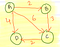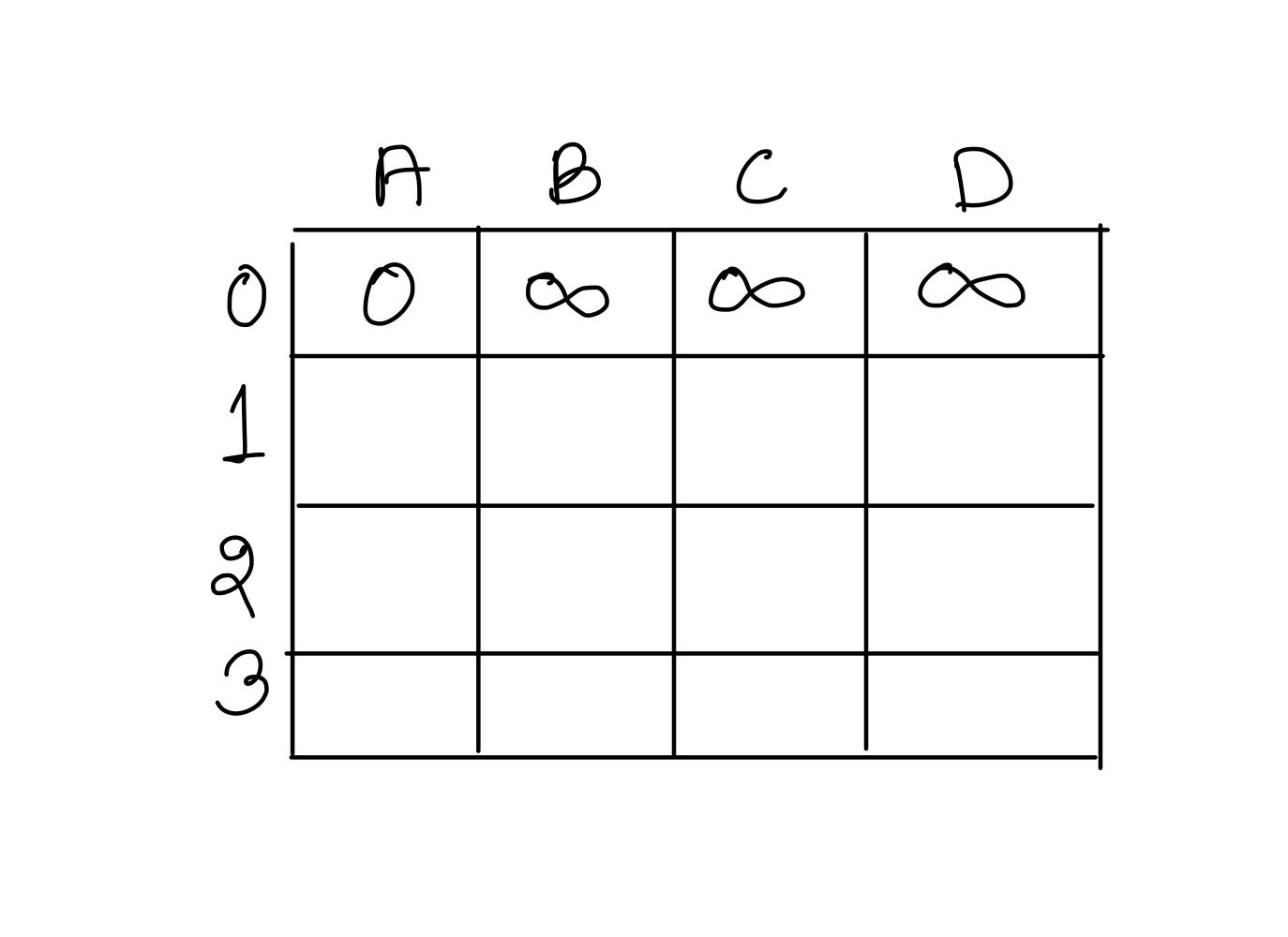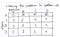# Bellman Ford’s Single Source Shortest Path algorithm from Dynamic Programming Perspective

Input: Given a weighted graph G with vertex set V and Edges E, a source vertex u ∈ V

V represents the set of all vertices, E is a collection of pairs (i,j) representing an edge from i to j

Problem: Find the weight of the shortest path from source vertex u to all the other vertices belonging to set V.

Shortest Path from source vertex u to any other vertex can have at most |V|-1 number of edges.

Given problem can be defined as:

if sp(i,e) represents weight of the shortest path from source vertex u to vertex i, which can have atmost e number of edges, then for all the vertices i ∈ V, find sp(i,|V|-1)

For example if the graph is:Example graph

Here if we treat A as the source vertex and try to find out the weight of the shortest path from A to all other vertices. Then we have to basically find out sp(B,3) sp(C,3) and sp(D,3).

## Optimal Substructure Property:

sp(i,e) can be expressed in terms of subproblems like this:

(x,i)∈E means for all the incoming edges to the vertex i , find out sp(x,e-1) + weight(x,i) which means weight of the shortest path from source vertex u to vertex x using e-1 number of edges + weight of the edge(x,i)

sp(i,e) = min ( for all incoming edges to the vertex i (x,i) { sp(x,e-1)+ weight of edge (x,i) } OR sp(i,e-1) )

So, if we were to find out the sp(C,3) in the above given graph.

Vertex C is having incoming edges from A and B. Therefore,

sp(C,3) = min ( (sp(A,2)+weight of (A,C)) OR (sp(B,2) + weight of edge(B,C)) OR sp(C,2) )

## Solution time

In reference to the graph taken above, to get the answer i.e sp(B,3), sp(C,3), sp(D,3). We start in bottom up manner i.e we first find out sp(B,1) sp(C,1) sp(D,1) then we try to find out sp(B,2) sp(C,2) sp(D,2) and then sp(B,3) sp(C,3) sp(D,3)

Initially, sp(C,0) = weight of shortest path from source vertex A to C using 0 edges which is infinity as there is no path from A to C using 0 edges. Similarly for others.Initial matrix

sp(C,1) = min( (sp(B,0)+weight(B,C)) OR (sp(A,0) + weight(A,C)) OR sp(C,0))

sp(C,1) = min( (INF + 3) OR (0 + 6) OR INF) = 6

Similarly, you can find sp(B,1) sp(D,1)

Now sp(C,2)= min( (sp(B,1)+weight(B,C)) OR (sp(A,1) + weight(A,C)) OR sp(C,1))

sp(c,2) = min( (2 + 3) OR (0+6) OR 6) = 5

Similarly, you can find out sp(B,2) sp(D,2)Filling up the matrix in row major order

Bellman Ford algorithm works fine for Graphs with negative edges as well.

## Time Complexity Analysis

Please note that while calculating sp(A,e), sp(B,e) sp(C,e) sp(D,e), it will take O(|E|) time. Because incoming edges of A + incoming edges of B + incoming edges of C + incoming edges of D = all the edges

So, finding out sp(A,e), sp(B,e), sp(C,e), sp(D,e) for e=0 to (|V|-1) times will take O(|V|*|E|).

Therefore time complexity of Bellman Ford’s Algorithm is O(|V|*|E|).

## Space Complexity Analysis:

Although the solution discussed above is utilizing O(|V|²) space. But it can be optimized to take only O(2*|V|) space because while calculating sp(i,e) we are taking the help of only (e-1) row.

Therefore space complexity of bellman ford algorithm is O(|V|).